CAT  >  Test Level 3: Ratio & Proportion

# Test Level 3: Ratio & Proportion

Test Description

## 10 Questions MCQ Test Level-wise Tests for CAT | Test Level 3: Ratio & Proportion

Test Level 3: Ratio & Proportion for CAT 2023 is part of Level-wise Tests for CAT preparation. The Test Level 3: Ratio & Proportion questions and answers have been prepared according to the CAT exam syllabus.The Test Level 3: Ratio & Proportion MCQs are made for CAT 2023 Exam. Find important definitions, questions, notes, meanings, examples, exercises, MCQs and online tests for Test Level 3: Ratio & Proportion below.
Solutions of Test Level 3: Ratio & Proportion questions in English are available as part of our Level-wise Tests for CAT for CAT & Test Level 3: Ratio & Proportion solutions in Hindi for Level-wise Tests for CAT course. Download more important topics, notes, lectures and mock test series for CAT Exam by signing up for free. Attempt Test Level 3: Ratio & Proportion | 10 questions in 20 minutes | Mock test for CAT preparation | Free important questions MCQ to study Level-wise Tests for CAT for CAT Exam | Download free PDF with solutions
 1 Crore+ students have signed up on EduRev. Have you?
Test Level 3: Ratio & Proportion - Question 1

### Two persons are awarded pensions in proportion to the square root of the number of years they have served. The second person's service period is 9 years longer than that of the first. If the length of service of the first person had exceeded that of the second by 4.25 years, their pensions would have been in the respective ratio of 9 : 8. How long have the two served?

Detailed Solution for Test Level 3: Ratio & Proportion - Question 1

Let n be the number of years served by the second.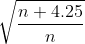=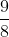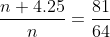64n + 272 = 81n
n = 16 years
First person's service = 16 - 9 = 7 years

Test Level 3: Ratio & Proportion - Question 2

### The monthly incomes of Vasu and Ravi are in the ratio 7 : 8 and their monthly expenditures are in the ratio 3 : 4, respectively. If the respective savings of Vasu and Ravi are Rs. 2,400 and Rs. 1,600, then find the monthly income of Ravi.

Detailed Solution for Test Level 3: Ratio & Proportion - Question 2

Ratio of monthly incomes of Vasu and Ravi = 7 : 8
Let their incomes be 7x and 8x, respectively.
Ratio of their expenditures = 3 : 4
Expenditure = Income - Savings
Savings of Vasu = Rs. 2,400
His expenditure = (7x - 2,400)
Savings of Ravi = Rs. 1,600
His expenditure = (8x - 1,600)
According to question: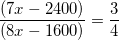28x - 9,600 = 24x - 4,800
4x = 4,800
x = 1,200
Monthly income of Ravi = 8x = (8 × 1,200) = Rs. 9,600

Test Level 3: Ratio & Proportion - Question 3

### Suman began a business with a capital of Rs. 50,000 and after 3 months, Manu entered into the partnership with a capital of Rs. 75,000. Three months later, Amandeep joined the firm with a capital of Rs. 1,25,000. At the end of the year, the firm made a profit of Rs. 99,495. How much of this sum did Amandeep receive?

Detailed Solution for Test Level 3: Ratio & Proportion - Question 3

Money invested by Suman for 12 months = Rs. 50,000
Money invested by Manu for 9 months = Rs. 75,000
Money invested by Amandeep for 6 months = Rs. 1,25,000
Share of Suman : Manu : Amandeep
= 50,000 × 12 : 75,000 × 9 : 1,25,000 × 6 = 6,00,000 : 6,75,000 : 7,50,000
= 600 : 675 : 750 = 8 : 9 : 10
Total profit = Rs. 99,495
Profit of Amandeep =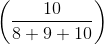× 99,495 =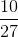× 99,495 = Rs. 36,850

Test Level 3: Ratio & Proportion - Question 4

The only people in each of the auditoriums A and B in a school are students, and each student in each of the auditoriums A and B is either from Grade 8 or from Grade 7. The ratio of number of students from Grade 8 to those from Grade 7 in auditorium A is 4 : 5. The ratio of number of students from Grade 8 to those from Grade 7 in auditorium B is 3 : 17, and the ratio of the total number of students from Grade 8 in both auditoriums to the total number of students from Grade 7 in both auditoriums A and B is 5 : 7. What is the ratio of the total number of students in auditorium A to the total number of students in auditorium B?

Detailed Solution for Test Level 3: Ratio & Proportion - Question 4

Let number of students of Grade 7 in auditorium A = 5a
Thus, number of students of Grade 8 in auditorium A = 4a
Let number of students of Grade 7 in auditorium B = 17b
Thus, number of students of Grade 8 in auditorium B = 3b
Total number of students of Grade 8 = 4a + 3b
Total number of students of Grade 7 = 17b + 5a
Now, (4a + 3b)/(17b + 5a) = 5/7
Or, a = (64/3)b
Let b = 3c
We get a = 64c
Total number of students in auditorium A = 9a = 576c
Total number of students in auditorium B = 20b = 60c
Required ratio = 576 : 60 = 48 : 5
Hence, answer option 4 is correct.

Test Level 3: Ratio & Proportion - Question 5

Three friends count the number of coins they have. They found that the ratio of the total number of coins of 25 p, 50 p and Re 1 is 11 : 6 : 13. They have 25 p coins in the ratio 2 : 4 : 5, 50 p coins in the ratio 6 : 3 : 9 and Re 1 coins in the ratio 7 : 2 : 4. Find the amount with the second person, if they have money only in the denomination of 25 p, 50 p and Re 1 and the total number of 25 p coins with them is 132.

Detailed Solution for Test Level 3: Ratio & Proportion - Question 5

Let the total number of coins = x
Total number of 25 coins = 132
The ratio of 25 p, 50 p and Re 1 coins is 11 : 6 : 13.
So, 25 p coins = x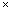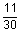132 = xx == 360
So, the total number of coins = 360
Total 50 p coins = 360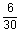= 72
Total 1 Re coins = 360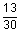= 156
Number of 25 p coins with second person = 132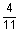= 48
Number of 50 p coins with second person = 72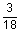= 12
Number of 1 Re coins with second person = 156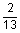= 24
That is, the second person have 48 coins of 25 p, 12 coins of 50 p and 24 coins of 1 Re.
Total money = 48 x 0.25 + 120.50 + 241 = 12 + 6 + 24 = Rs. 42

Test Level 3: Ratio & Proportion - Question 6

A balloon can go up in the air at the rate of 24 kmph and its speed diminishes by a quantity, which varies as the square root of the number of stones attached to it. With four stones, its speed is 20 kmph. The highest number of stones with which the balloon can go up in the air is

Detailed Solution for Test Level 3: Ratio & Proportion - Question 6

Diminishing speed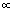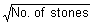Let speed be S1 when no stones are attached.
S1 = 24
Let S2 be the speed when stones are attached (where N be the number of stones)
S2 = 24 -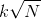(where k is proportionality constant)
According to given condition;
20 = 24 -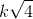2k = 4
k = 2
S= 24 -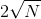For S2 = 0 , we have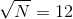N = 144
It means that if we tie 144 stones, the balloon cannot go up in the air.
So, the highest number of stones that can be attached with the balloon is 143.

Test Level 3: Ratio & Proportion - Question 7

Tanya and Sanya invested some amount of money in the ratio 7 : 6 for the same period. At the end of the year, 20% of the profit was donated to an old age home. Out of the remaining profit, 50% is reinvested and the rest is divided in the ratio of their initial contribution. If the difference between their shares of profit is Rs. 4400, what was the total profit?

Detailed Solution for Test Level 3: Ratio & Proportion - Question 7

Let the total profit be Rs. x.
After donating 20% profit to old age home, remaining profit = 0.8x.
Out of the remaining profit, 50% is reinvested.
So, the remaining profit = 0.4x
Now this 0.4x has to be divided in the ratio 7 : 6.
Tanya's share = 0.4x ×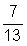Sanya's share = 0.4x ×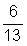Difference = 0.4x ×– 0.4x ×= 4400
= 0.4x ×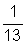= 4400
x =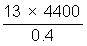= Rs. 1,43,000

Test Level 3: Ratio & Proportion - Question 8

Three persons Akbar, Birbal and Tansen invested in a business Rs. 10,000, Rs. 25,000 and Rs. P, respectively. Akbar withdrew Rs. 1,000 after 3 months and Rs. 1000 again after 5 months. Birbal invested Rs. 7,000 more than his initial investment after 9 months. Tansen maintained his capital for the whole year. If at the end of the year, they made a profit of Rs. 12,400, and Tansen got Rs. 2,400 as his share in the profit, find the initial investment made by Tansen.

Detailed Solution for Test Level 3: Ratio & Proportion - Question 8

Total investment made by Akbar = 10,000 × 3 + 9,000 × 2 + 8,000 × 7
= 30,000 + 18,000 + 56,000 = 1,04,000
Total investment made by Birbal = 25,000 × 9 + 32,000 × 3
= 2,25,000 + 96,000 = 3,21,000
Total investment made by Tansen = P × 12
Total profit = 12,400
Share of Tansen in total profit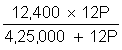2,400 =2,400 × 4,25,000 + 2,400 × 12P = 12,400 × 12P
2,400 × 4,25,000 = 10,000 × 12P
P =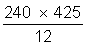= 8,500
So, initial investment made by Tansen = Rs. 8,500

Test Level 3: Ratio & Proportion - Question 9

Mr. Alaram designed a new clock, which has 12 divisions as usual, represented by the numbers 1 to 12. But the speed of the minute hand is directly proportional to the digit, which it is going to approach, i.e. if the minute hand is between 1 and 2, its speed is proportional to 2 and if it is between 11 and 12, its speed is proportional to 12. The speed of the minute hand, when it is between 12 and 1 is equal to that of an ordinary clock and both the clocks were set at 9 : 00 am.

What will be the time in the ordinary clock, if the new clock shows 9 : 30 am?

Detailed Solution for Test Level 3: Ratio & Proportion - Question 9

Since, in between 12 and 1, the speed of minutes hand is similar to that of an ordinary clock, it take 5 minutes to cover first division.
Then its speed will become twice, because, the hand is between 1 and 2.
So, it takes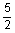minutes. Between 2 and 3, the speed becomes thrice and takes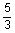minutes.
So, in total it takes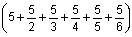minutes to reach 6 (9 : 30 am).
= 5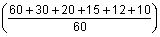=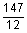minutes = 12¼ minutes.
So, ordinary clock shows 9 hours 12 minutes 15 seconds.

Test Level 3: Ratio & Proportion - Question 10

Amounts deposited by two friends Harpreet and Gagan are in the ratio 7 : 9. Total amount deposited is Rs. 72,000. After some time, Harpreet withdraws Rs. 7500. Find the money withdrawn by Gagan, so that the new ratio of their deposits may become 4 : 5.

Detailed Solution for Test Level 3: Ratio & Proportion - Question 10

Amount deposited by Harpreet and Gagan = Rs. 72,000
Amounts are in the ratio 7 : 9.
Let common ratio = x
Money deposited by Harpreet = 7x
Money deposited by Gagan = 9x
Now, 7x + 9x = Rs. 72,000
16x = Rs. 72,000
x = Rs. 4500
Money deposited by Harpreet = 4500 × 7 = Rs. 31,500
Money deposited by Gagan = 4500 × 9 = Rs. 40,500
Money left with Harpreet after withdrawal of Rs. 7500 = 31,500 - 7500 = Rs. 24,000
Ratio of amounts of Harpreet and Gagan is 4 : 5.
So, amount of Gagan = 5/4 × 24,000 = Rs. 30,000
Amount withdrawn by Gagan = 40,500 - 30,000 = Rs. 10,500

## Level-wise Tests for CAT

5 docs|272 tests
 Use Code STAYHOME200 and get INR 200 additional OFF Use Coupon Code
Information about Test Level 3: Ratio & Proportion Page
In this test you can find the Exam questions for Test Level 3: Ratio & Proportion solved & explained in the simplest way possible. Besides giving Questions and answers for Test Level 3: Ratio & Proportion, EduRev gives you an ample number of Online tests for practice

## Level-wise Tests for CAT

5 docs|272 tests

### How to Prepare for CAT

Read our guide to prepare for CAT which is created by Toppers & the best Teachers